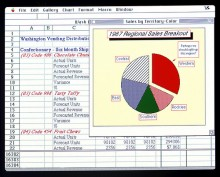# How Do I Calculate a Percentage In a Excel Worksheet Using a Formula?The easiest way to lay this out and calculate is to break the sums down in to a number of cells.

First put your initial figure in to a cell - here I will use the first one available but you can change it based on the arrangement of your spreadsheet. So 3000 goes in to cell A1.

In the next cell, in this instance cell A2, put your second figure of 7.50. In the third cell, A3, don't input the answer. Instead insert a formula. This will mean you can adjust the first two factors and the calculation will be done for you.

To insert the first formula, click on the cell A3 and type =A1*A2. This means calculate the figure in cell A1 multiplied by the figure in cell A2. The answer will automatically appear in the cell A3.

Next we can calculate what 1 per cent of the figure in A3 is. In your next cell, A4, type =A3/100. This tells the spreadsheet to divide the figure in the cell A3 by 100.

The next step is to show what 8 per cent of the figure is. This is easy. Create a new cell, I'll use A5. Type the formula =A4*8. The computer will multiply the figure in A4 by 8 and put the answer in the A5 cell. So A5 will show you what 8 per cent of your initial figure of 22,500 is.

Finally we can produce a total in the final cell, A6. To do so we need to show the result of the first calculation with the figure of 8per cent added to it. Therefore in the cell type =A3+A5.

So from your question and following it through as above, the spreadsheet cells will read as follows:
A1 - 3000
A2 - 7.5
A3 - 22,500
A4 - 225
A5 - 1687.5
A6 - 24187.5

There are faster ways to show this on the spreadsheet using more detailed formulas in fewer cells. This is the easiest way though as it shows a series of simple calculations. The benefit of doing it this way is that if you change the value in the cell A1 or A2, all of the other values will change and you can visible break down what it means.
thanked the writer.